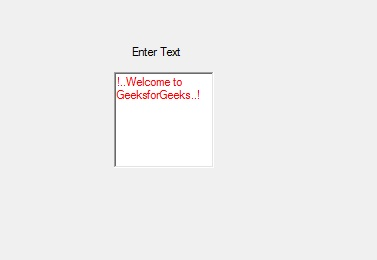Related Articles
How to set the Location of the RichTextBox in C#?
• Last Updated : 17 Jul, 2019

In C#, RichTextBox control is a textbox which gives you rich text editing controls and advanced formatting features also includes a loading rich text format (RTF) files. Or in other words, RichTextBox controls allows you to display or edit flow content, including paragraphs, images, tables, etc. In RichTextBox, you are allowed to set the location of the RichTextBox control in the windows forms using Location Property. This property contains the coordinates of the upper-left corner of the RichTextBox control relative to the upper-left corner of its form. You can set this property in two different ways:

1. Design-Time: It is the easiest way to set the location of the RichTextBox as shown in the following steps:

• Step 1: Create a windows form as shown in the below image:
Visual Studio -> File -> New -> Project -> WindowsFormApp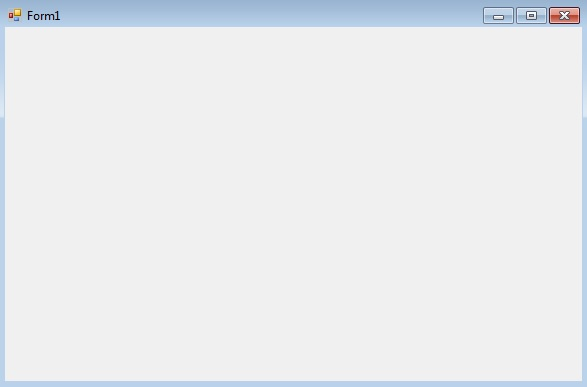• Step 2: Drag the RichTextBox control from the ToolBox and drop it on the windows form. You are allowed to place a RichTextBox control anywhere on the windows form according to your need.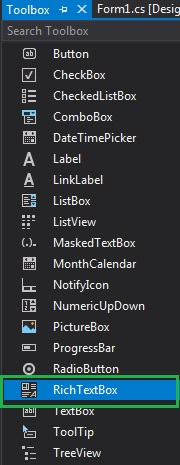• Step 3: After drag and drop you will go to the properties of the RichTextBox control set the location of the RichTextBox control.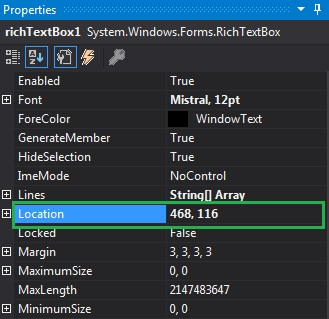Output: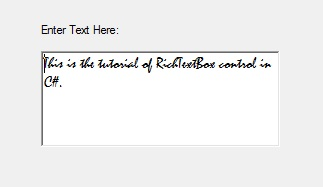2. Run-Time: It is a little bit trickier than the above method. In this method, you can set the location of the RichTextBox control programmatically with the help of given syntax:

`public System.Drawing.Point Location { get; set; }`

Here, the Point indicates the upper-left corner of the RichTextBox control relative to the upper-left corner of its form. The following steps show how to set the Location property of the RichTextBox dynamically:

• Step 1: Create a RichTextBox using the RichTextBox() constructor is provided by the RichTextBox class.
```// Creating RichTextBox using RichTextBox class constructor
RichTextBox rbox = new RichTextBox();
```
• Step 2: After creating RichTextBox, set the Location property of the RichTextBox provided by the RichTextBox class.
```// Setting the location of the RichTextBox
rbox.Location = new Point(236, 97);
```
• Step 3: And last add this RichTextBox control to the form using Add() method.
```// Add this RichTextBox to the form
 `using` `System;``using` `System.Collections.Generic;``using` `System.ComponentModel;``using` `System.Data;``using` `System.Drawing;``using` `System.Linq;``using` `System.Text;``using` `System.Threading.Tasks;``using` `System.Windows.Forms;`` ` `namespace` `WindowsFormsApp30 {`` ` `public` `partial` `class` `Form1 : Form {`` ` `    ``public` `Form1()``    ``{``        ``InitializeComponent();``    ``}`` ` `    ``private` `void` `Form1_Load(``object` `sender, EventArgs e)``    ``{``        ``// Creating and setting the ``        ``// properties of the label``        ``Label lb = ``new` `Label();``        ``lb.Location = ``new` `Point(251, 70);``        ``lb.Text = ``"Enter Text"``;`` ` `        ``// Adding this label in the form``        ``this``.Controls.Add(lb);`` ` `        ``// Creating and setting the ``        ``// properties of the RichTextBox``        ``RichTextBox rbox = ``new` `RichTextBox();``        ``rbox.Location = ``new` `Point(236, 97);``        ``rbox.ForeColor = Color.Red;``        ``rbox.Text = ``"!..Welcome to GeeksforGeeks..!"``;`` ` `        ``// Adding this RichTextBox in the form``        ``this``.Controls.Add(rbox);``    ``}``}``}`